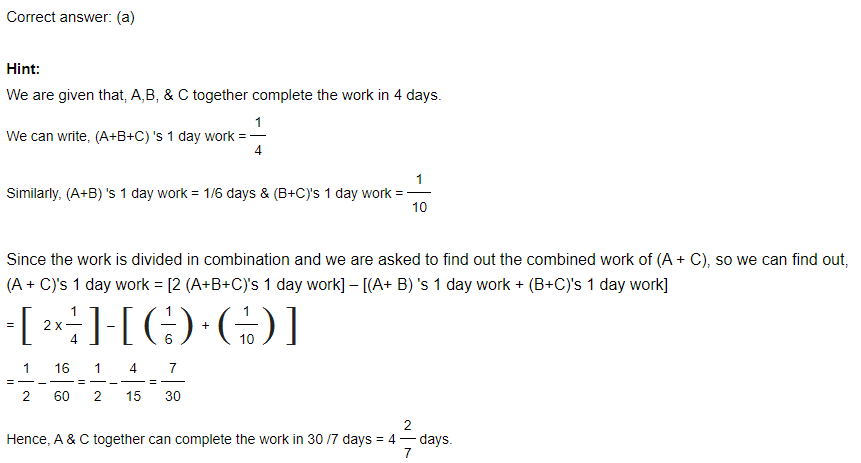# Dedicated for NMAT 2023# Time & Work NMAT 2023Assume that Pooja completes the job in ‘x’ days.
So, Aarti will take ‘2x’ days to complete the same job.

As Pooja takes 90 days less than Aarti, we get
x = 2x – 90
By solving this equation, we get x = 90 .
Thus, 2x = 2 x 90 = 180

Part of job done by Pooja in 1 day = 1/ 90
Part of job done by Aarti in 1 day = 1/180

(Part of job done together in 1 day) = (Part of job done by Pooja in 1 day) + (Part of job done by Aarti in 1 day)
= (1/90) + (1/180)
= 3/180
=1/60
(1/60)th part of whole job will be completed by Pooja and Aarti together in one day.

Therefore, the whole job will be completed in 60 days together.

Hint: If ‘A’ is ‘x’ times as good a workman as ‘B’, then ratio of work done by A & B = x: 1
Monika’s 1 day work : Sonika’s 1 day work = 2:1
(Monika’s + Sonika’s ) 1 day work = 1 /20
Divide 1/20 in the ratio 2:1 ———( To divide the number ‘a’ into ratio x & y , we have first part = ax / x + y )

Assume 1 man’s 1 day work = x & 1 boy’s 1 day work = y
From the given data, we can generate the equations as : 4x + 5y = 1/20 —(1) & 5x + 4y = 1/16 —(2)
By solving the simultaneous equations (1) & (2),
x = 1/ 80 & y = 0

#### Popular Post

• ###### Time Speed Distance in NMAT 2023Lorem ipsum dolor sit amet consectetur adipiscing elit ut elit tellus luctus nec.

## Pricing Plans For Every Need

Lorem ipsum dolor sit amet consectetur adipiscing elit semper dalar elementum tempus hac tellus libero accumsan dolor sit amet consctur.## 2000/-

Best books for NMAT Exam!

12 Yellow Books

Full Syllabus

Focus on Vocab Grammar

Critical Reasoning

Quant All topics covered

DI & DS intensive prep## 2500/-

Best Shortcuts for NMAT Exam

Shortcuts for Quant

G Strategy for DI DS

Vocab Grammar G Strategy

Logical Reasoning Shortcuts

Live Classes and Recordings

Weekly Targets## 2000/-

Best Mocks for NMAT Exam